# Gravitational lens

File:GravitationalLensingScenario.png
When the gravitational field of an object - the gravitational lens - deflects passing light rays, this can cause an observer to see multiple images of a single source.

In a gravitational lensing scenario, light traveling from a distant astronomical source (e.g. a galaxy) to an observer is deflected by the gravitational field of an intermediate object: the gravitational lens (e.g. a cluster of galaxies). Because of this, the observer will see the source in a slightly different direction and if the gravitational field is strong enough, light rays may reach the observer by multiple paths, thereby generating several images of the same source.

##  History

File:GravitationalDeflectionAngle.png
The angle$\hat{\alpha}$ is called the deflection angle.

In Newtonian mechanics, the path of a test particle in a gravitational field does not depend on the the particle's mass. For this reason, one can argue that even in the absolute space and time of Newton, light does not always go in a straight line, but can be deflected noticeably by objects with a sufficiently strong gravitational field. In 1804, J. von Soldner was the first one to publish the Newtonian value of the deflection angle (as illustrated in the figure on the right):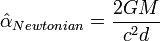$\hat\alpha_{Newtonian} = \frac{2 G M}{c^2 d}$

In this equation, G is the gravitational constant, M the mass of the object deflecting the light, c is the speed of light and d is the distance of closest approach.

More than a century later, after having completed his general theory of relativity, Albert Einstein published a new expression, differing by a factor of two from the Newtonian result: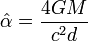$\hat\alpha = \frac{4 G M}{c^2 d}$

For a light ray passing close to the Sun, the value of this angle is 1.74 seconds of arc. During a solar eclipse, the bending angle can be calculated by comparing the positions of stars close to the sun to the positions where one would expect these stars to be seen if their light rays were not deflected by the sun. In 1919, a team of astronomers led by Eddington set out to measure the deflection angle during a total eclipse and reported an agreement with Einstein's predicted value with an uncertainty measure of 20%. Later measurements drastically reduced this amount of uncertainty to a value of 0.02%.

After this, gravitational deflection of light received only little attention for a very long time. Around 1964 Liebes and especially Refsdal revived the gravitational lens effect briefly, but it wasn't until the first actual gravitationally lensed quasar QSO 0957+561 was observed in 1979 that the subject gained much interest. Since then, a huge amount of articles about gravitational lensing have been published.

##  Regimes

Gravitational lens phenomena are usually subdivided into three categories: strong lensing, weak lensing and microlensing. In the strong lensing regime, the gravitational lens effect causes multiple images of the same source to be observed. Often, there are severely distorted images such as giant arcs. For strong lensing to occur, the source, the gravitational lens and the observer have to be sufficiently well aligned and the bending angle has to be large enough to allow the different images to be resolved. Some strong lensing examples include:

• SDSS J1004+4112: here, a cluster of galaxies acts as a gravitational lens, producing five images of a distant quasar and three of a distant galaxy.
• G2237+0305: a galaxy causes four images of a distant quasar to appear. This gravitational lens is often referred to as the Einstein Cross.
• CL 0024+1654: a cluster of galaxies (yellow) creates several images of a distant galaxy (blue).

In some cases the alignment is not well enough to produce multiple images, but the effect of the gravitational lens can still be seen through the presence of a multitude of slightly distorted images. This is normally referred to as weak lensing. Since it is impossible to know the exact shape of a source a priori, a statistical analysis of a large number of images is necessary to acquire information about the distortions caused by the gravitational lens.

Finally, it is possible that the gravitational lens does cause multiple images, but that these simply cannot be resolved by our telescopes. However, since a gravitational lens can cause images to become brighter, the presence of a gravitational lens can be detected when source and lens move with respect to each other. In this case, the gravitational lens effect is referred to as microlensing.

##  Lensing formalism

To describe the gravitational lens effect, usually the lensing formalism described below is employed. This framework is only valid if the angles involved are small and if the gravitational field is not too strong. It can be applied to lensing by stars, galaxies and clusters of galaxies, but not to strong deflection by a black hole for example.

###  Deflection angle

To derive the deflection angle predicted by general relativity, one usually starts from a space-time continuum described by the static weak-field metric, which has the following line element:$\mathrm{d}s^2 = \left(1+\frac{2\Phi}{c^2}\right)\mathrm{d}t^2-\left(1-\frac{2\Phi}{c^2}\right)(\mathrm{d}x^2+\mathrm{d}y^2+\mathrm{d}z^2)$.

Here, Φ is the Newtonian gravitational potential of the lensing mass. It can be shown that the path of a light ray in this metric is mathematically equivalent to the path of a light ray in Newton's absolute space and time if there's a location-dependent refractive index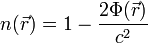$n(\vec{r}) = 1-\frac{2\Phi(\vec{r})}{c^2}$.

Fermat's theorem from geometric optics says that light goes from source to destination using paths for which the corresponding time delay is extremal. Applying this principle to calculate the deflection angle for a point mass leads to Einstein's expression:$\hat{\alpha} = \frac{4 G M}{c^2 d}$.

###  Lens equation

Since the distances involved in gravitational lensing are enormous compared to the distance scale at which the deflection takes place, the mass distribution of the gravitational lens is approximated as a two-dimensional one, located in the so-called lens plane. If the z-axis lies along the source-lens direction, this two-dimensional mass distribution Σ can be obtained from the three-dimensional distribution ρ as follows:$\Sigma(\vec{\xi}) = \int\rho(\xi_1,\xi_2,z)\mathrm{d}z$,

where$\vec{\xi}$ is a vector in the lens plane.

Light rays are then assumed to travel in a straight line from the source to the lens plane. At this point, they are deflected instanteneously and continue along a straight line towards the observer. The situation is illustrated in the figure below. Projecting the path of a light ray (thick black line) onto the xz and yz planes shows the two angles$\hat{\alpha}_x$ and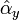$\hat{\alpha}_y$ needed to describe the gravitational deflection; these are the components of a vector-based bending angle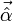$\vec{\hat{\alpha}}$. Based on Einstein's expression for the bending angle due to a point mass, the gravitational deflection of an arbitrary two-dimensional mass distribution Σ can easily be seen to be: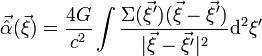$\vec{\hat{\alpha}}(\vec{\xi}) = \frac{4 G}{c^2}\int \frac{\Sigma(\vec{\xi}')(\vec{\xi}-\vec{\xi}')}{|\vec{\xi}-\vec{\xi}'|^2} \mathrm{d}^2 \xi'$.

Similar to the concept of the lens plane, the source is said to lie in the so-called source plane. If Dd, Ds and Dds are observer-lens, observer-source and lens-source distances respectively, one can write the following expression for a light ray going from a source plane point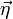$\vec{\eta}$ to the observer, while being deflected at lens plane point$\vec{\xi}$: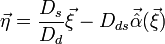$\vec{\eta} = \frac{D_s}{D_d}\vec{\xi}-D_{ds}\vec{\hat{\alpha}}(\vec{\xi})$,

where we used our initial assumption that the angles involved are small. The figure below illustrates the situation. Introducing the direction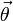$\vec{\theta}$ and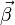$\vec{\beta}$, where$D_d \vec{\theta} = \vec{\xi}$ and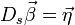$D_s \vec{\beta} = \vec{\eta}$, one can re-write the previous expression to obtain the lens equation or ray-trace equation:$\vec{\beta}(\vec{\theta}) = \vec{\theta}-\frac{D_{ds}}{D_s}\vec{\hat{\alpha}}(\vec{\theta})$.

For the lens equation to be correct on a cosmological scale as well, the distance measures used have to be interpreted as angular diameter distances, for which in general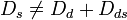$D_s \ne D_d + D_{ds}$.

The lens equation relates a direction$\vec{\theta}$ to a corresponding direction$\vec{\beta}$. Basically what it means is the following: when looking in a direction described by$\vec{\theta}$, one sees what would be seen in direction$\vec{\beta}(\vec{\theta})$ if the gravitational lens were absent. The$\vec{\theta}$-space describes what images can be seen due to the gravitational lens effect and is often referred to as the image plane. The$\vec{\beta}$-space is usually identified with the source plane, as it describes what would be seen without the gravitational lens.

If the shape and position of the source is known as well as the mass distribution of the lens, a simple procedure can be used to calculate the images produced by the gravitational lens effect. For a large number of points in the image plane, the corresponding positions in the source plane are calculated. The points which lie inside the source are detected and the corresponding image plane points are a part of an image of the source. Effectively, one traces rays of light from the observer back to the source plane, which is why the lens equation is also often called the ray trace equation. This procedure is necessary since only for some very simple mass distributions the lens equation can be inverted to immediately obtain the image plane positions for a specific source plane position.

###  Brightness of the images

File:GravitationalLensMagnification.png
The deflection of light rays by a gravitational lens can affect the flux received by an observer, causing some images to be brighter and some to be dimmer.

The figure on the right illustrates how a gravitational lens can affect the flux received by an observer. In the image, an observer uses a detector of area A. If the gravitational lens were absent, the observer would receive the photons emitted in a relatively small solid angle, indicated by the dashed lines. However, in this example the presence of the lens causes photons emitted in a much larger solid angle to hit the detector, causing the image to be brighter. Of course, in a similar way it is possible for images to become dimmer as well. Since the difference between the angles involved is very small, in practice one can ignore the possible anisotropic radiation of light rays.

The magnification factor μ describes how much brighter or dimmer an image of a point source becomes. One can show that if the lens equation is known, this factor can be calculated as follows: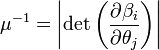$\mu^{-1} = \left|\mathrm{det}\left(\frac{\partial \beta_i}{\partial \theta_j}\right)\right|$,

i.e. the inverse magnification factor equals the absolute value of the Jacobian determinant of the transformation described by the lens equation.

As the Jacobian determinant above approaches zero, the magnification factor becomes infinitely large, causing very bright images. The points in the image plane for which this is the case, together form the so-called critical lines. Using the lens equation one can calculate the corresponding curves in the source plane, called the caustics. The figure on the right shows the critical lines (red) and caustics (blue) for a gravitational lens with an elliptical mass distribution. It also shows the five images of a circular source at the center. The mass distribution itself is not shown.

The position of a source relative to the caustics determines the number of images produced. If a source is outside all caustics, there will be only a single image. Each time a source crosses a caustic, two new images are produced. Therefore, in general a gravitational lens always creates an odd number of images. In the figure on the right, one has to cross two caustics to reach the source, each time adding two images to obtain a total number of five images.

In practice, of course, there is no such thing as an infinitely bright image. This can already be resolved if one takes into account the fact that a source is never a true point source, but has some finite extension. However, even a point source would not have an infinite magnification. In the regions where the magnification becomes large, one would have to use wave optics to calculate the true effect, instead of using the less accurate geometric optics.

###  Time delay

For a source position described by$\vec{\beta}$ one can consider all possible paths from source to observer and the actual paths light rays will follow are then determined by Fermat's principle. In our approximation the only paths considered are those which go straight from the source to the lens plane and afterwards go in a straight line towards the source. The time delay for a light ray reaching the observer from a direction$\vec{\theta}$ is then described by the time delay function: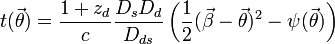$t(\vec{\theta}) = \frac{1+z_d}{c}\frac{D_s D_d}{D_{ds}}\left(\frac{1}{2}(\vec{\beta}-\vec{\theta})^2-\psi(\vec{\theta})\right)$.

In this expression, zd is the cosmological redshift of the gravitational lens and ψ is the effective lensing potential or projected potential which, in terms of$\vec{\xi}$ is defined as follows:$\psi(\vec{\xi}) = \frac{D_{ds}}{D_s D_d}\frac{2}{c^2} \int \Phi(\xi_1,\xi_2,z) \mathrm{d}z$,

where Φ again is the Newtonian gravitational potential. It can be shown that the gradient of the projected potential is related to the deflection angle:$\vec{\nabla}_\vec{\theta} \psi = \frac{D_{ds}}{D_s}\vec{\hat{\alpha}}$.

Applying Fermat's principle to calculate the image positions from the time delay function means one has to find its stationary points. This means calculating$\vec{\nabla}_\vec{\theta}t = 0$ which immediately yields the lens equation.

##  Applications

###  Cosmic telescope

As is mentioned above, a gravitational lens can have a serious effect on how the observer perceives an astronomical object. It is possible that one of the images (or several images) are magnified, making them easier to observe. Because of this, a gravitational lens can make very distant objects visible, which would otherwise be far to dim to observe. For this reason, gravitational lenses are sometimes referred to as cosmic telescopes.

Abell 2218 is a cluster of galaxies acting as a gravitational lens for several background objects. Thanks to its magnifying effect, an extremely distant galaxy has been observed.

###  Detection of planets

File:GravitationalMicroLensingCurve.png
Typical shape of a brightness curve in a microlensing event

When an object moves with respect to another star acting as a gravitational lens, this will produce a typical brightness curve such as the one on the right. If a planet orbits the star acting as a gravitational lens, it is possible that the measured brightness curve reveals the presence of this planet by introducing an additional small bump for example. The advantage of this planet detection method is that it permits the discovery of planets having masses of only a small fraction of the star's mass. A handful of planets has already been detected this way. 

###  Lens inversion

When you know where the source would be in absence of a gravitational lens and the mass distribution of the lens is known as well, it is relatively easy to calculate the images caused by the gravitational lens effect. Of course, in practice you neither know the position of the source nor the exact mass distribution of the lens. Instead we face the inverse problem: if we know the images caused by the gravitational lens effect, what is the mass distribution which makes them appear.

Lens-inversion techniques are usually subdivided into two classes. Parametric methods use a specific model for the mass distribution, for example an elliptical one. Using the images of one or more sources as input, the inversion routine then tries to find the parameters of the lens (position, ellipticity, rotation) which fit these images best. Non-parametric methods do not assume a particular shape of the mass distribution. One way to do this is to assume a mass distribution to be the sum of a large number of basis functions, the weights of which are determined by an inversion routine.

All matter present in the gravitational lens contributes to the gravitational bending of light rays. Because of this, gravitational lens inversion provides an independent probe of the mass of a galaxy or a cluster of galaxies. If the mass density retrieved in this way is compared to the visible amount of matter, this provides a great deal of information about the presence of dark matter.

###  Cosmological parameters

Because of the deflection of light by a gravitational lens, light rays from a source can reach an observer using multiple paths. The time it takes light to travel from source to observer is in general different for each path. If the source is one of varying brightness, measuring the brightness curves for each image for a while can reveal information about the relative time delays. It is easy to see that the expansion of the universe influences the time it takes light to go from source to observer. The Hubble constant describes this rate of expansion and for this reason, the relative time delays between the images of a source can provide constraints on the value of the Hubble constant.

The gravitational lens effect can also supply information about other cosmological parameters. For example, statistical information about the occurrence of gravitationally lensed object can provide insight into the values of the matter density and the cosmological constant.Some content on this page may previously have appeared on Citizendium.